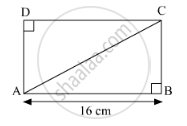Share

# Find the Diagonal of a Rectangle Whose Length is 16 Cm and Area is 192 Sq.Cm. - Geometry

ConceptApplication of Pythagoras Theorem in Acute Angle and Obtuse Angle

#### Question

Find the diagonal of a rectangle whose length is 16 cm and area is 192 sq.cm ?

#### SolutionIt is given that, area of rectangle is 192 sq.cm.

$\text{Area} = \text{Length} \times \text{Breadth}$
$\Rightarrow 192 = 16 \times \text{BC}$
$\Rightarrow \text{BC} = \frac{192}{16}$
$\Rightarrow \text{BC} = 12 \text{cm} . . . \left( 1 \right)$

According to Pythagoras theorem,

In ∆ABC

${\text{AB}}^2 + {\text{BC}}^2 = {\text{AC}}^2$
$\Rightarrow \left( 16 \right)^2 + \left( 12 \right)^2 = {\text{AC}}^2$
$\Rightarrow 256 + 144 = {\text{AC}}^2$
$\Rightarrow {\text{AC}}^2 = 400$
$\Rightarrow \text{AC} = 20 \text{cm}$

Hence, the length of a diagonal of the rectangle is 20 cm.

Is there an error in this question or solution?

#### APPEARS IN

Balbharati Solution for Balbharati Class 10 Mathematics 2 Geometry (2018 to Current)
Chapter 2: Pythagoras Theorem
Problem Set 2 | Q: 4 | Page no. 44

#### Video TutorialsVIEW ALL 

Solution Find the Diagonal of a Rectangle Whose Length is 16 Cm and Area is 192 Sq.Cm. Concept: Application of Pythagoras Theorem in Acute Angle and Obtuse Angle.
S